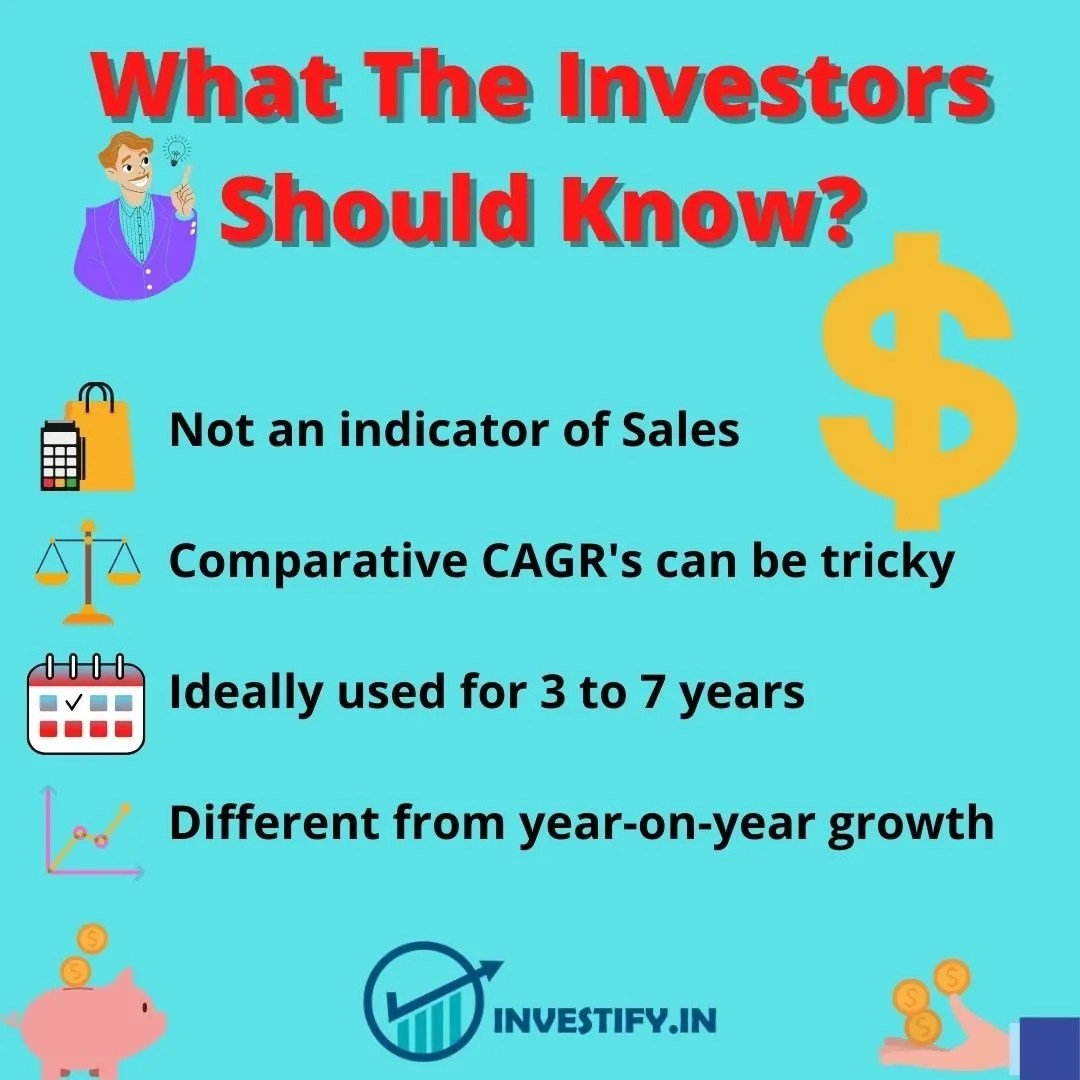CAGR! Seems like a complicated name, isn’t it? This stands for compounded annual growth rate. Thus, it is actually a wonderful phenomenon. Knowingly or unknowingly, we all have come across it. In the earlier decades, our parents used to deposit money in the post offices. The reason was that it was subsequently profitable and compound interest was involved. Interest on interest and thus your invested money will fetch a higher return eventually. We hereby seek to simplify this concept for our readers. Let’s begin!

### What is CAGR?

CAGR is the full form of Compound Annual Grow Rate. Also, CAGR is the return on investment an investor gets from the beginning(also known as an acquisition) to the end(also known as a sale). This is based on the assumption that all the profits have been reinvested.

However, it also exceeds the significance that is given to annual return. The higher return in a year can be due to an extraordinary gain. This can be owing to multiple factors. Nonetheless, the investor should look for consistent returns and make his choices accordingly as CAGR reflects the average returns an investor would get.

Traditionally, CAGR is used to measure the past performance of any investment or predict their future returns. Moreover, it gives the most precise results for calculating the returns on assets, portfolios, or any other securities. This is because their value can increase or decrease with time.

In simple terms, CAGR signifies how much an investment has grown on an average in a year. It is expressed in percentage. For example, consider an investor who wants to invest in an ELSS scheme with a 5-year tenure. CAGR in this case will represent the extent of returns that the fund gave to the investor every year during this period. This is only applicable if the returns are reinvested.

### How is CAGR calculated?

Investors rely on absolute returns. They should also consider the time value of money. CAGR gives an approximate growth rate for an investment. The investment should be free from volatility.

Analyzes past performance or predicts the future returns.

Let’s understand the CAGR formula from the 3 points,

1. The initial investment made at the starting year.

2. The final value of an investment in the final year.

3. Duration of the investment.

### Formula for CAGR

CAGR = (Final Value/Initial Value)^(1/Years) – 1

Let’s suppose, an investor had bought a stock for Rs 100 in 2010. In 2011, the value of the stock increased to Rs 125. In 2012, it finally became Rs 150. Thus, as you can see, there is a 50% total increase in value. If you want to know the annual growth rate for this investment, use the compound annual growth rate! Using the above formula, the CAGR for 2010 to 2012 will be 22.47.

This formula will also predict the future value of the investment. Hence, it will make it easier to compare the values of investment and the predicted price.### What the Investors should know?

1. Not an indicator of sales: Compound annual growth rate is not an indicator of sales from the beginning to the end of the year. Growth focused in initial or end years.

2. Comparative CAGRs can be tricky: Two CAGRs can be the same, while one might be more attractive than the other. The reason can be thus because one might have experienced good growth initially, while the other might have experienced good growth in the end.

3. Ideally used for 3 to 7 years: Best suited for 3 to 7 years investments. For investments in excess of 10 years, CAGR tends to hide the sub-trend.

4. Different from year-on-year growth: Compound annual growth rate is different from year-on-year growth rate(as of 31 March 2019 vs 31 March 2020).

1. A compound annual growth rate is one of the most accurate methods of predicting the return on investment as the investment will have a fall and rise in its value over the investment period.

2. Helps investors in analyzing different time periods of investment.

3. Allows risk-free instruments to calculate the returns from any particular investment.### Limitations

1. The compound annual growth rate does not consider investment risk.

2. It does not factor in uncertainty. It does not reflect the phases of volatility the investment has been through.

3. CAGR does not measure the profitability of an asset with inflows and outflows during the investment period. It only measures the return rate on an investment based on the initial and final price.

### Applications of CAGR formula

CAGR finds its usage in the following scenarios,

1. In the current year, you have purchased certain units of an equity fund. This has subsequently increased the value of your fund. CAGR will help you estimate the returns on your investment.

2. Suppose an investor is willing to invest with certain goals and objectives. With the CAGR formula, they will be able to predict the rate at which their money will grow.

3. If you are planning to invest in an equity fund with 3,5 and 10-year returns of 30%,18%, and 12% respectively, CAGR can help you determine the average annual growth rate.

4. Comparing the compound annual growth rate of a mutual fund with benchmark return can tell about the performance of the fund in the market.

### Conclusion

Compound annual growth rate helps in determine the compounded growth rate, especially in a mutual fund which an investor will receive after a certain time. It thus helps in determining a mutual fund scheme's average annual growth rate. It helps in finding out the performance of a stock over a period of time, how much an investor has lost or gained. However, it also has its limitations as it does not take into account the volatility in the markets. It remains a reliable tool to measure the performance of any stock and helps you to make calculated decisions on your investments.